# Chapter 13 Linear Equations in Two Variables RD Sharma Solutions Exercise 13.4 Class 9 Maths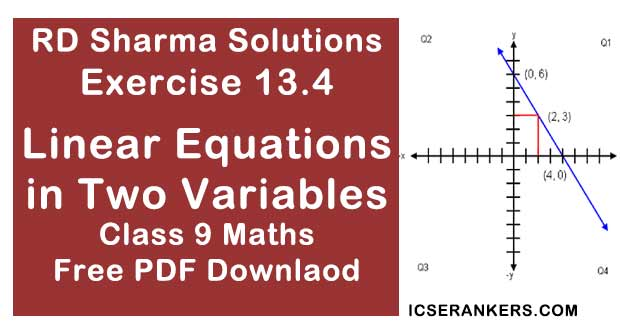Chapter Name RD Sharma Chapter 13 Linear Equations in Two Variables Exercise 13.4 Book Name RD Sharma Mathematics for Class 10 Other Exercises Exercise 13.1Exercise 13.2Exercise 13.3 Related Study NCERT Solutions for Class 10 Maths

### Exercise 13.4 Solutions

1. Given the geometric representations of the following equations
(a) on the number line
(b) on the Cartesian plane :
(i) x = 2

(ii) y + 3 = 0

(iii) y = 3

(iv) 2x+  9 = 0

(v) 3x - 5 = 0

Solution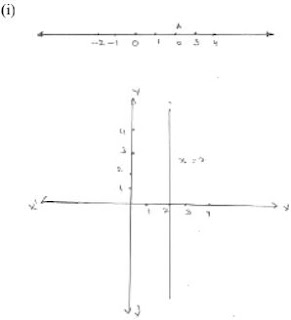x = 2
Point A represents x = 2 number line
On Cartesian plane, equation represents all points on y - axis for which x = 2

(ii)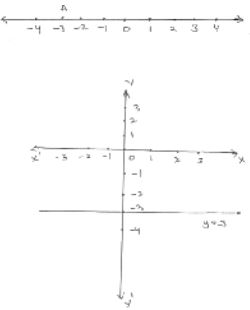y = 3
Point A represents 3 on number line
On Cartesian plane, equation represents all the points on x - axis for which y = -3 .

(iii)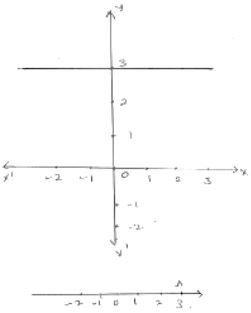y = 3.
Point A represents 3 on number line
On Cartesian plane, equation represents all points all points on x - axis for which y = 3

(iv)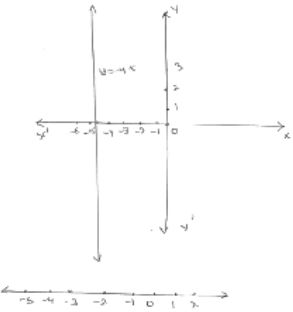2x + 9 = 0
2x = -9
x = -9/2 = -4.5
Point A represents -4.5 on number line
On Cartesian plane, equation represents all points on y - axis for which x = -4.5

(v)3x - 5 = 0
3x = 5
x = 5/3 = 1.6 (Approx)
Point A represents 1(1/2) or 5/3 on number line
On Cartesian plane, equation represents all points on y - axis for which x = 16

2. Give the geometrical representation of 2x + 13 = 0 as an equation in
(i) one variable
(ii) two variable
Solution
(i)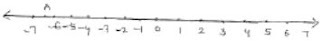One variable representation of 2x + 13 = 0
2x = -13
x = -13/2 = -6(1/2)
Points A represents  -13/2

(ii)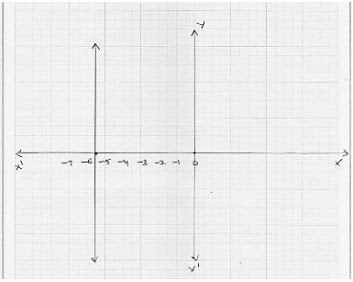Two variable representation of 2x + 13 = 0
2x + 0y + 13 =  0
⇒ 2x + 13 = 0
⇒ 2x = - 13
⇒ x = -13/2
⇒ x = -6.5
On Cartesian plane, equation represents all points y - axis for which x = -6.5.

3. Solve the equation 3x + 2 = x - 8, and represent the solution (i) the number line (ii) the Cartesian plane.
Solution
(i)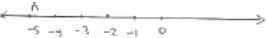3x + 2 = x - 8
⇒ 3x - x = -8 - 2
⇒ 2x = -10
⇒ x = -5
Points A represents -5 on number line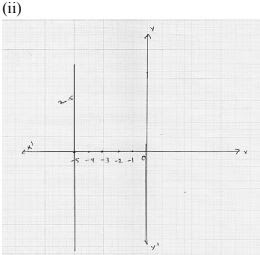On Cartesian plane, equation represents all points on y - axis for which x = 5

4. Write the equation of the line that is parallel to x - axis and passing through the point
(i) (0, 3)
(ii) (0, -4)
(iii) (2, -5)
(iv) (-4, - 3)
Solution
(i) The equation of the line that is parallel to x - axis and passing through the point (0, 3) is y = 3
(ii) The equation of the line that is parallel to x - axis and passing through the point (0, -4) is y = 4
(iii) The equation of the line that is parallel to x - axis and passing through the point (2, -5) is y = -5
(iv) The equation of the line that is parallel to x - axis and passing through the point (-4, - 3) is y = -3

5. Write the equation of the line that is parallel to y - axis and passing through the point
(i) (4, 0)
(ii) (-2, 0)
(iii) (3, 5)
(iv) (-4, -3)
Solution
(i) The equation of the line that is parallel to y - axis and passing through (4, 0) will be x = 4
(ii) The equation of the line that is parallel to y - axis and passing through (-2, 0) will be x = -2
(iii) The equation of the line that is parallel to y - axis and passing through (3, 5) will be x = 3
(iv) The equation of the line that is parallel to y- axis and passing through (-4, -3) will be x = -4.